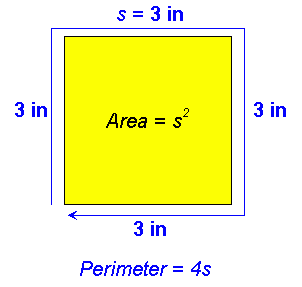From: Larry, a parent If the perimeter of a square is 1 foot, its area is how many square inches? The answer in the book is 9 inches to the second power. Please explain how to get this answer. Hi Larry. First, for our non-American readers, we need to remember that 12 inches = 1 foot. Here's a diagram I've drawn to help understand the question:Since a square has all sides the same length, the perimeter (the distance around the outside of an object) is four times the side length (let's call this s). You know that the perimeter is 12 inches (one foot), so if we divide that by four, we get each side is length 3 inches. Now we also know that a square's area is the side length times itself, that is: s2. So (3 in)2 is 9 in2 . Hope this explanation helps! Stephen La Rocque.